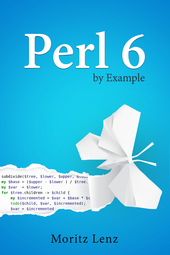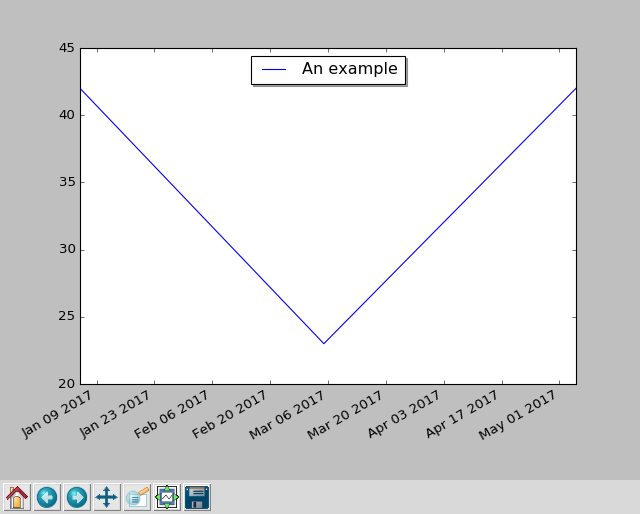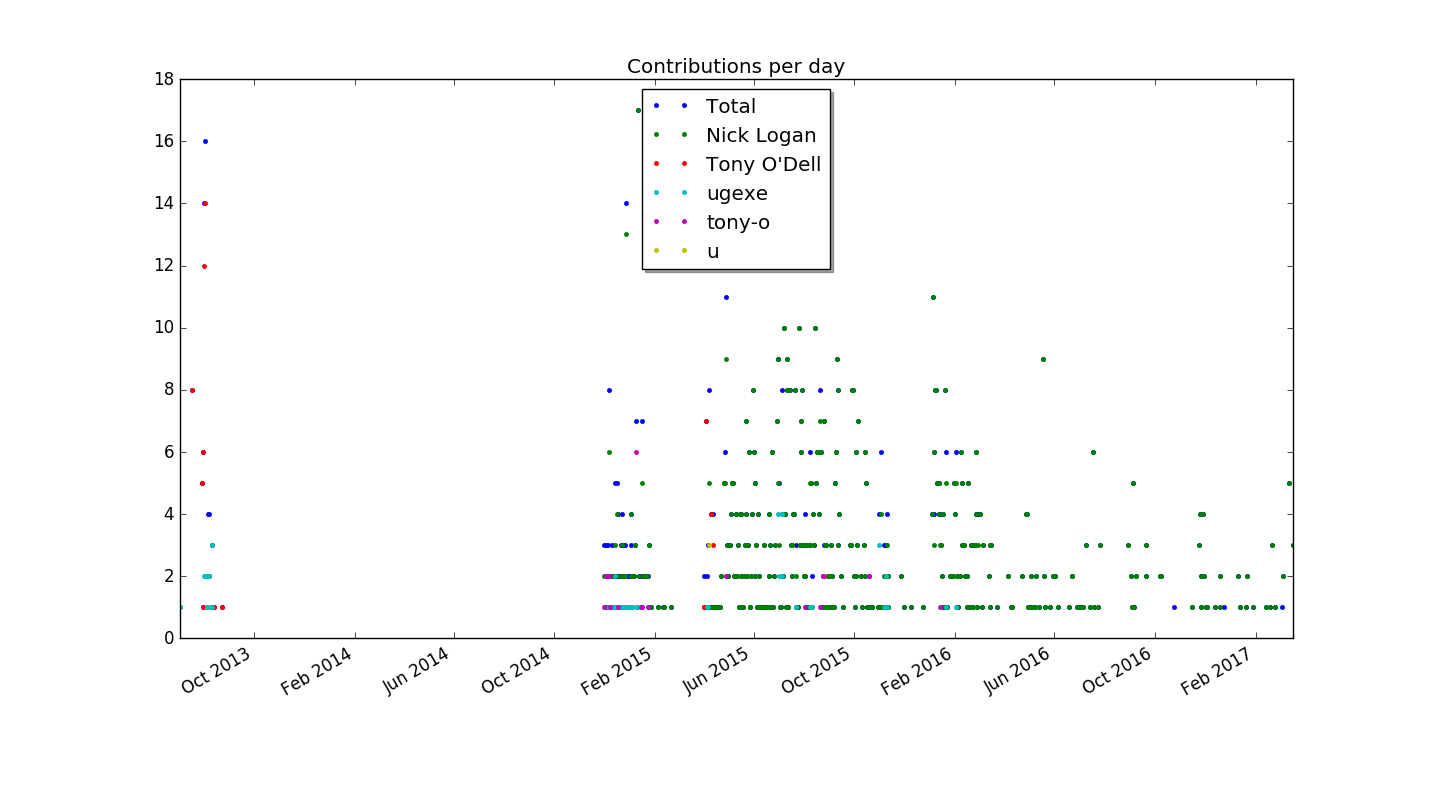# Perl 6 By Example: Plotting using Matplotlib and Inline::PythonThis blog post is part of my ongoing project to write a book about Perl 6.

If you're interested, either in this book project or any other Perl 6 book news, please sign up for the mailing list at the bottom of the article, or here. It will be low volume (less than an email per month, on average).

Occasionally I come across git repositories, and want to know how active they are, and who the main developers are.

Let's develop a script that plots the commit history, and explore how to use Python modules in Perl 6.

## Extracting the Stats

We want to plot the number of commits by author and date. Git makes it easy for us to get to this information by giving some options to `git log`:

``````my \$proc = run :out, <git log --date=short --pretty=format:%ad!%an>;
my (%total, %by-author, %dates);
for \$proc.out.lines -> \$line {
my ( \$date, \$author ) = \$line.split: '!', 2;
%total{\$author}++;
%by-author{\$author}{\$date}++;
%dates{\$date}++;
}
``````

`run` executes an external command, and `:out` tells it to capture the command's output, and makes it available as `\$proc.out`. The command is a list, with the first element being the actual executable, and the rest of the elements are command line arguments to this executable.

Here `git log` gets the options `--date short --pretty=format:%ad!%an`, which instructs it to print produce lines like `2017-03-01!John Doe`. This line can be parsed with a simple call to `\$line.split: '!', 2`, which splits on the `!`, and limits the result to two elements. Assigning it to a two-element list `( \$date, \$author )` unpacks it. We then use hashes to count commits by author (in `%total`), by author and date (`%by-author`) and finally by date. In the second case, `%by-author{\$author}` isn't even a hash yet, and we can still hash-index it. This is due to a feature called autovivification, which automatically creates ("vivifies") objects where we need them. The use of `++` creates integers, `{...}` indexing creates hashes, `[...]` indexing and `.push` creates arrays, and so on.

To get from these hashes to the top contributors by commit count, we can sort `%total` by value. Since this sorts in ascending order, sorting by the negative value gives the list in descending order. The list contains Pair objects, and we only want the first five of these, and only their keys:

``````my @top-authors = %total.sort(-*.value).head(5).map(*.key);
``````

For each author, we can extract the dates of their activity and their commit counts like this:

``````my @dates  = %by-author{\$author}.keys.sort;
my @counts = %by-author{\$author}{@dates};
``````

The last line uses slicing, that is, indexing an array with list to return a list elements.

## Plotting with Python

Matplotlib is a very versatile library for all sorts of plotting and visualization. It's written in Python and for Python programs, but that won't stop us from using it in a Perl 6 program.

But first, let's take a look at a basic plotting example that uses dates on the `x` axis:

``````import datetime
import matplotlib.pyplot as plt

fig, subplots = plt.subplots()
subplots.plot(
[datetime.date(2017, 1, 5), datetime.date(2017, 3, 5), datetime.date(2017, 5, 5)],
[ 42, 23, 42 ],
label='An example',
)
fig.autofmt_xdate()
plt.show()
``````

To make this run, you have to install python 2.7 and matplotlib. You can do this on Debian-based Linux systems with `apt-get install -y python-matplotlib`. The package name is the same on RPM-based distributions such as CentOS or SUSE Linux. MacOS users are advised to install a python 2.7 through homebrew and macports, and then use `pip2 install matplotlib` or ```pip2.7 install matplotlib``` to get the library. Windows installation is probably easiest through the conda package manager, which offers pre-built binaries of both python and matplotlib.

When you run this scripts with `python2.7 dates.py`, it opens a GUI window, showing the plot and some controls, which allow you to zoom, scroll, and write the plot graphic to a file:## Bridging the Gap

The Rakudo Perl 6 compiler comes with a handy library for calling foreign functions, which allows you to call functions written in C, or anything with a compatible binary interface.

The Inline::Python library uses the native call functionality to talk to python's C API, and offers interoperability between Perl 6 and Python code. At the time of writing, this interoperability is still fragile in places, but can be worth using for some of the great libraries that Python has to offer.

To install `Inline::Python`, you must have a C compiler available, and then run

``````\$ zef install Inline::Python
``````

(or the same with `panda` instead of `zef`, if that's your module installer).

Now you can start to run Python 2 code in your Perl 6 programs:

``````use Inline::Python;

my \$py = Inline::Python.new;
\$py.run: 'print("Hello, Pyerl 6")';
``````

Besides the `run` method, which takes a string of Python code and execute it, you can also use `call` to call Python routines by specifying the namespace, the routine to call, and a list of arguments:

``````use Inline::Python;

my \$py = Inline::Python.new;
\$py.run('import datetime');
my \$date = \$py.call('datetime', 'date', 2017, 1, 31);
\$py.call('__builtin__', 'print', \$date);    # 2017-01-31
``````

The arguments that you pass to `call` are Perl 6 objects, like three `Int` objects in this example. `Inline::Python` automatically translates them to the corresponding Python built-in data structure. It translate numbers, strings, arrays and hashes. Return values are also translated in opposite direction, though since Python 2 does not distinguish properly between byte and Unicode strings, Python strings end up as buffers in Perl 6.

Object that `Inline::Python` cannot translate are handled as opaque objects on the Perl 6 side. You can pass them back into python routines (as shown with the `print` call above), or you can also call methods on them:

``````say \$date.isoformat().decode;               # 2017-01-31
``````

Perl 6 exposes attributes through methods, so Perl 6 has no syntax for accessing attributes from foreign objects directly. If you try to access for example the `year` attribute of `datetime.date` through the normal method call syntax, you get an error.

``````say \$date.year;
``````

Dies with

``````'int' object is not callable
``````

Instead, you have to use the `getattr` builtin:

``````say \$py.call('__builtin__', 'getattr', \$date, 'year');
``````

## Using the Bridge to Plot

We need access to two namespaces in python, `datetime` and `matplotlib.pyplot`, so let's start by importing them, and write some short helpers:

``````my \$py = Inline::Python.new;
\$py.run('import datetime');
\$py.run('import matplotlib.pyplot');
sub plot(Str \$name, |c) {
\$py.call('matplotlib.pyplot', \$name, |c);
}

sub pydate(Str \$d) {
\$py.call('datetime', 'date', \$d.split('-').map(*.Int));
}
``````

We can now call `pydate('2017-03-01')` to create a python `datetime.date` object from an ISO-formatted string, and call the `plot` function to access functionality from matplotlib:

``````my (\$figure, \$subplots) = plot('subplots');
\$figure.autofmt_xdate();

my @dates = %dates.keys.sort;
\$subplots.plot:
\$[@dates.map(&pydate)],
\$[ %dates{@dates} ],
label     => 'Total',
marker    => '.',
linestyle => '';
``````

The Perl 6 call `plot('subplots')` corresponds to the python code `fig, subplots = plt.subplots()`. Passing arrays to python function needs a bit extra work, because `Inline::Python` flattens arrays. Using an extra `\$` sigil in front of an array puts it into an extra scalar, and thus prevents the flattening.

Now we can actually plot the number of commits by author, add a legend, and plot the result:

``````for @top-authors -> \$author {
my @dates = %by-author{\$author}.keys.sort;
my @counts = %by-author{\$author}{@dates};
\$subplots.plot:
\$[ @dates.map(&pydate) ],
\$@counts,
label     => \$author,
marker    =>'.',
linestyle => '';
}

plot('title', 'Contributions per day');
plot('show');
``````

When run in the zef git repository, it produces this plot:## Summary

We've explored how to use the python library matplotlib to generate a plot from git contribution statistics. `Inline::Python` provides convenient functionality for accessing python libraries from Perl 6 code.

In the next installment, we'll explore ways to improve both the graphics and the glue code between Python and Perl 6.

## Subscribe to the Perl 6 book mailing list

* indicates required